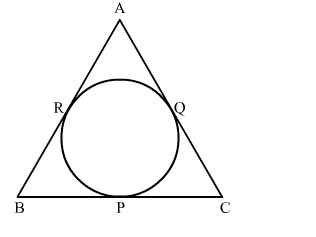# In the given figure, an isosceles triangle ABC with AB = AC, circumscribes a circle.Question:

In the given figure, an isosceles triangle ABC with AB = AC, circumscribes a circle. Prove that the point of contact P bisects the base BCSolution:

We know that tangent segments to a circle from the same external point are congruent.
Now, we have
AR = AQ, BR = BP and CP = CQ
Now, AB = AC
⇒ AR + RB = AQ + QC
⇒ AR + RB = AR + QC
⇒ RB = QC
⇒ BP = CP
Hence, P bisects BC at P.